####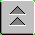Damage Control Training Stability and Buoyancy Lessons

LESSON TOPIC: 4.9 TITLE: ENHANCED FLOODING EFFECTS DIAGRAM PREPARATION AND UNDERWAY DRAFT REPORT

Contact periods allotted this LESSON TOPIC:

Classroom: 2.0 Test: 0.0

Trainer: 0.0 Total: 2.0

TERMINAL OBJECTIVES:

6.0 EVALUATE shipboard stability by analyzing weight and moment considerations (JTI 2.1, 3.2.1, 6.0, 6.1, 6.2)

ENABLING OBJECTIVES:

6.54 Given a ship's DC book, section II(a), booklet of general plans, and/or subdivision diagram, PREPARE stability data cards for ship's compartments.

6.55 Given stability data cards, PREPARE a flooding effects diagram enhanced with: weight added, changes to drafts forward and aft, sine correction and list.

6.56 Given sample fuel/water reports, CALCULATE underway drafts using the Trim Calculation sheet.

6.57 Given Trim Calculation sheets, COMPLETE an underway draft report.

STABILITY DATA CARD AND FLOODING EFFECTS

DIAGRAM PREPARATION

INTRODUCTION: This lesson will introduce you to a technique of accurately and rapidly determining your ship's stability situation after flooding damage. This method is based on the fact that 90% of the necessary calculations can be performed prior to actual damage, within a reasonable degree of accuracy.

REFERENCES: Damage Control Book Section II(a)

Booklet of General Plans for Your Ship

Stability Student Guide Lesson 4.9

MATERIALS: 1. 2 Stability Data Cards

2. 1 Blank Flooding Effects Worksheet

3. Calculator

4. Booklet of General Plans for your Ship

6. Subdivision Diagram

7. Damage Control Book

8. Ruler

9. Dividers

10. Markers: Yellow, Green, and Pink

JOB STEPS: 1. Select 2 WATERTIGHT compartments to prepare stability data cards for. DO NOT select tanks which are included on the liquid loading diagram. Choose one centerline compartment and one off-centerline or asymmetrical compartment. Compartments must be surrounded by watertight boundaries. NOTE: This consideration causes a group of compartments separated by joiner bulkheads and doors to be treated as one. This concept is accurate; when one space floods, all spaces within the subdivision will flood. DO NOT pick two compartments which are identical (i.e opposing wing tanks).

2. Prepare stability data cards for these 2 compartments completing the 25% and 100% columns. Take accurate measurements from the Booklet of General Plans for length, width, and height, as well as height above the keel. (If Booklet of General Plans is not available, use Watertight Subdivision Diagram, DC Diagram #2, and estimate values as best you can.)

3. Sketch the 2 selected compartments in their approximate locations on the flooding effects worksheet. (see page 4.9.12 for example)

JOB STEPS: 4. Write the compartment number, or numbers, in the center of each compartment.

5. From the stability data card 100% flooded column, write the number of tons added in the upper left hand corner of each compartment.

6. Directly below tons added, write the sine correction for 100% (two decimal places) for each compartment.

7. In the upper right hand corner, write the change in list caused by flooding to 100%.

8. In the bottom left hand corner, write the change in drafts aft (to the nearest inch) for each compartment.

9. In the bottom right hand corner, write the change in drafts forward (to the nearest inch) for each compartment.

10. COLOR THE COMPARTMENTS in accordance with the flooding effects diagram table below:

 COLOR SINE COR 25% SINE COR 100% PINK + + YELLOW + — GREEN — — WHITE < 0.01 < 0.01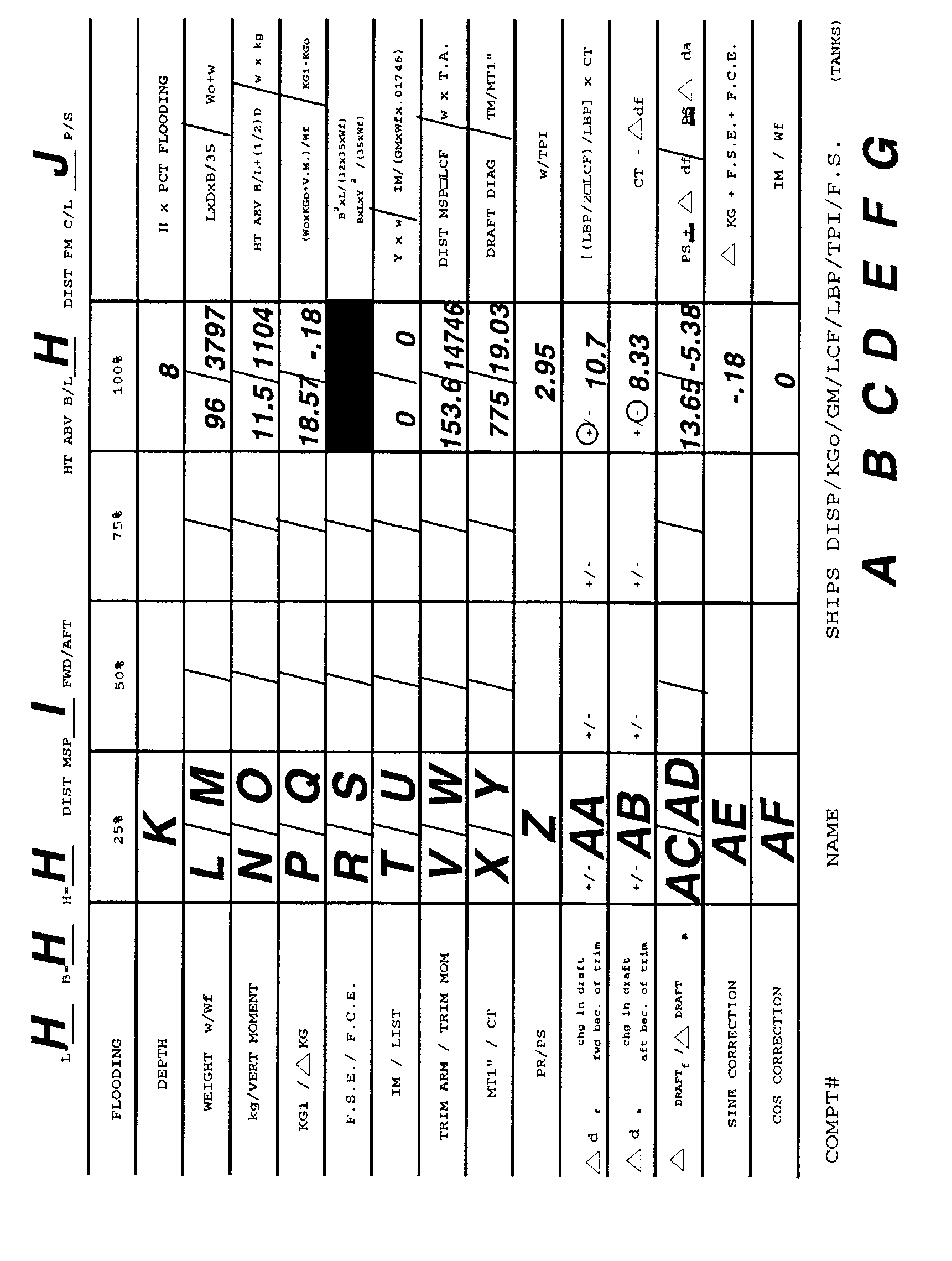COMPARTMENT STABILITY DATA CARD

The Stability Data Card calculates the effect on ship stability when flooding a particular compartment. Prior to damage, the change in KG due to weight addition, free surface effect, free communication effect, and also changes in trim can be calculated. This information can be printed on standard 8½ x 11 paper and kept on file. A card should be filled out for each compartment below the waterline.

To accurately complete this form, the DCA will need the BOOKLET OF GENERAL PLANS, DAMAGE CONTROL BOOK, a pair of dividers and a calculator.

 BLOCK PROCEDURE A W0 Full Load Displacement (DC Book) B KG0 Height of the Center of Gravity for Full Load Condition (DC Book) C GM Metacentric Height, uncorrected for Free Surface Effect (DC Book) D LCF Longitudinal Center of Flotation (Draft Diagram and Functions of Form) E LBP Length Between Perpendiculars (DC Book) F TPI Tons Per Inch Immersion at Full Load (Draft Diagram and Functions of Form) G F.S.(TANKS) Free Surface Effect in Ship's Tanks at Full Load (DC Book) H L Length, B Breadth, H Height of the compartment and the HT ABV B/L height of the DECK of the compartment above the keel (Booklet of General Plans) I DIST MSP Distance from Mid-Ships Perpendicular to the center of the compartment. Circle FWD if the center of the compartment is forward of Mid-Ships, circle AFT if it is aft (Booklet of General Plans) J DIST FM C/L Distance from the CENTER of the compartment to the centerline of the ship. Circle P if the compartment is on the port side, and S if on the starboard side (Booklet of General Plans) K Depth Depth of flooding in feet. Multiply the height of the compartment by the percentage flooding. BLOCK PROCEDURE L Weight Weight added. Use the equation: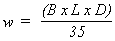M WF Final displacement. Use the equation: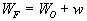N kg Height of the center of gravity of the flooding above the keel. Use the equation: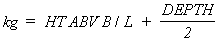O Vert Moment Use the equation: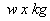P KG1 Use the equation: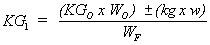Q DKG The difference between KG0 and the KG1. If KG1 is less than KG0 DKG will be negative. It is important that the (+/-) be recorded on the form. R F.S.E. Free Surface Effect. Use the equation: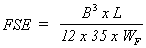NOTE: When flooding is 100%, Free Surface = 0.00 FT S F.C.E. Free Communication Effect. Use the equation: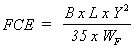T IM Inclining moment. Use the equation: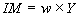where Y is (DIST FM C/L P/S) U LIST Angle of list. Use the equation: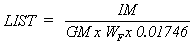V TRIM ARM Trimming arm. Use the equation: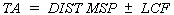W TRIM MOM Trimming moment. Use the equation: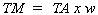BLOCK PROCEDURE X MT1" Moment to Trim One Inch. (Draft Diagram and Function of Form) Use WF as the entering argument. Because WF will vary with the level of compartment flooding, MT1" for 25% and 100% will not be the same. Y CT Change in Trim. Use the equation: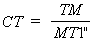Z PR/PS Parallel rise/parallel sinkage. Use the equation: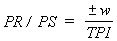AA DdF Change in draft forward due to trim. Use the equation: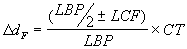NOTE: If the trimming moment is forward, DdF is positive. If the trimming moment is aft, DdF is negative. Circle the appropriate sign on the stability data card. AB DdA Change in draft aft due to trim. Use the equation: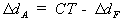NOTE: If the trimming moment is forward, DdA is negative. If the trimming moment is aft, DdA is positive. Circle the appropriate sign on the stability data card. AC DDRAFTF Total change in draft forward. Change in draft at the bow due to parallel rise/sinkage and trim. Use the equation: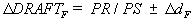AD DDRAFTA Total change in draft aft. Change in draft at the stern due to parallel rise/sinkage and trim. Use the equation: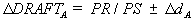AE SINE CORRECTION The total change in the height of the center of gravity. Use the equation: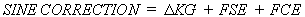AF COSINE CORRECTION The distance the center of gravity is shifted off-centerline. Use the equation: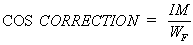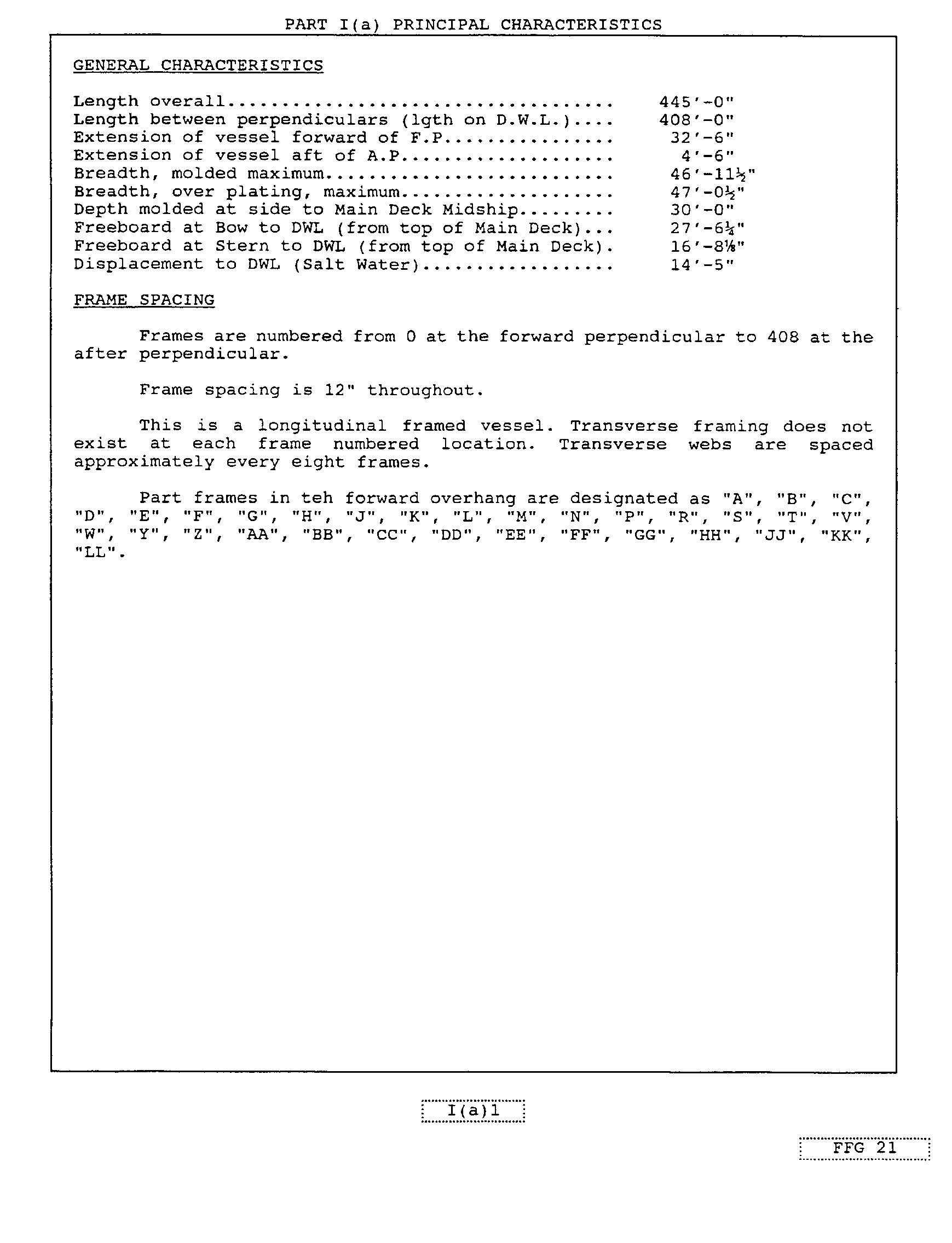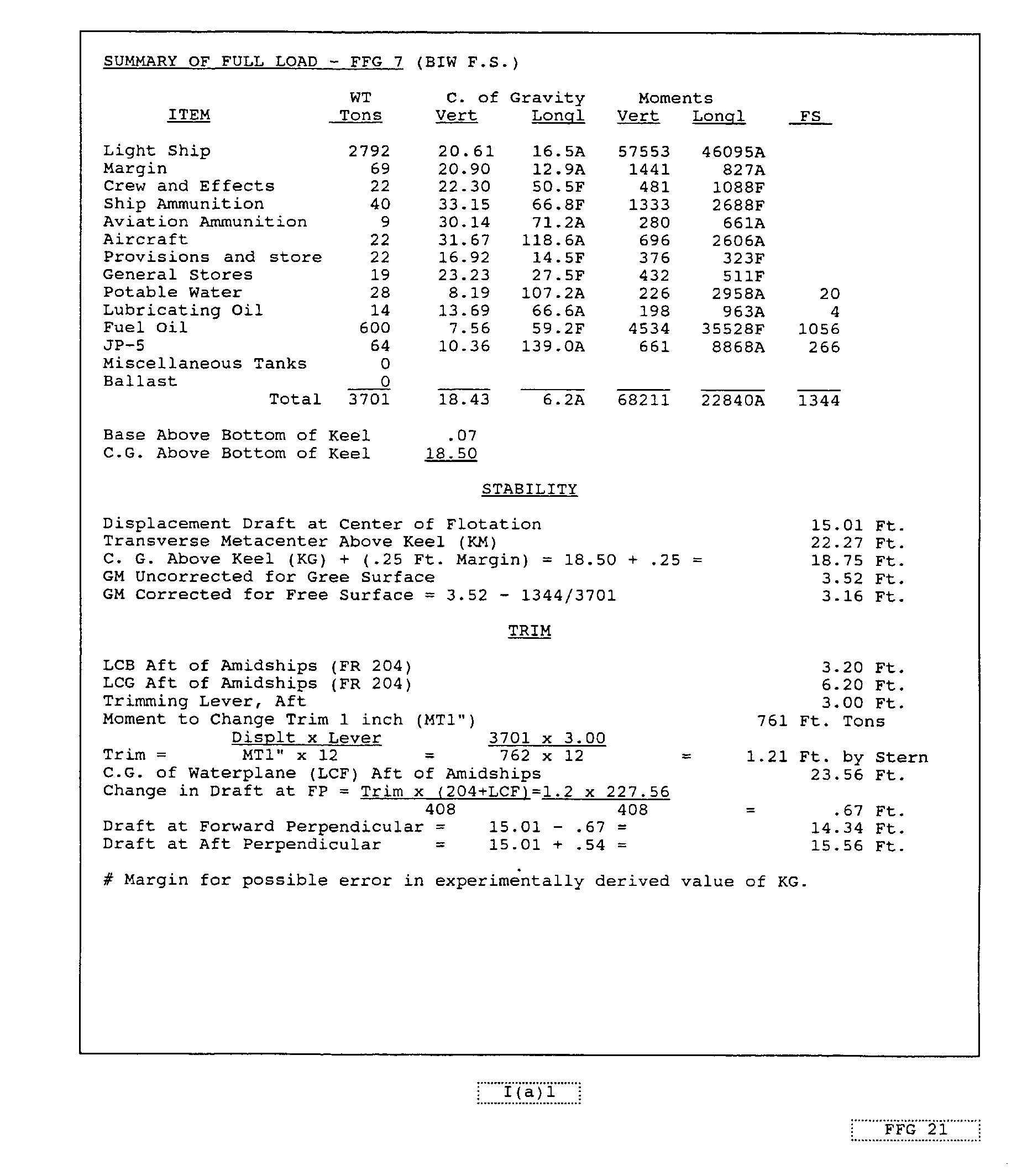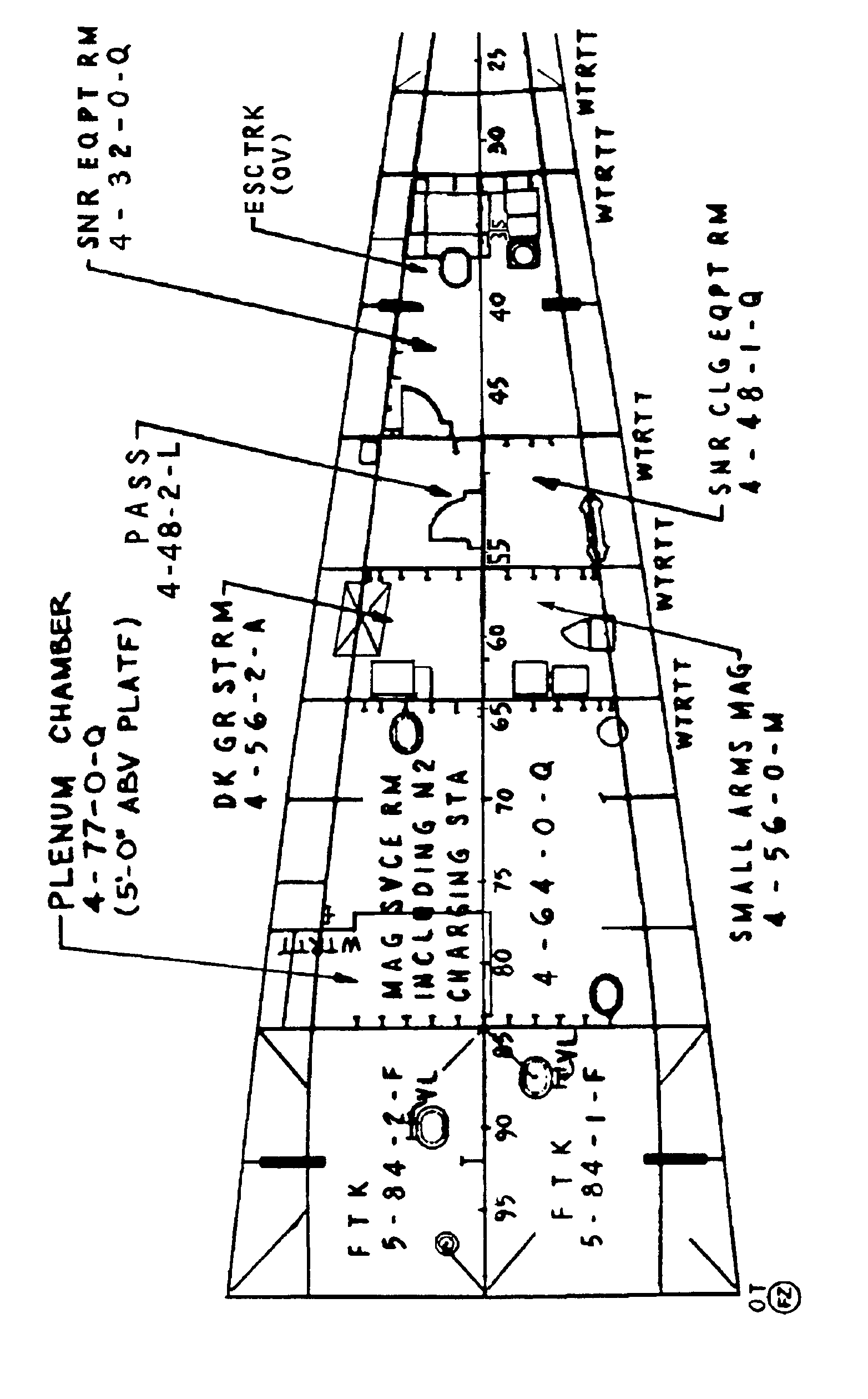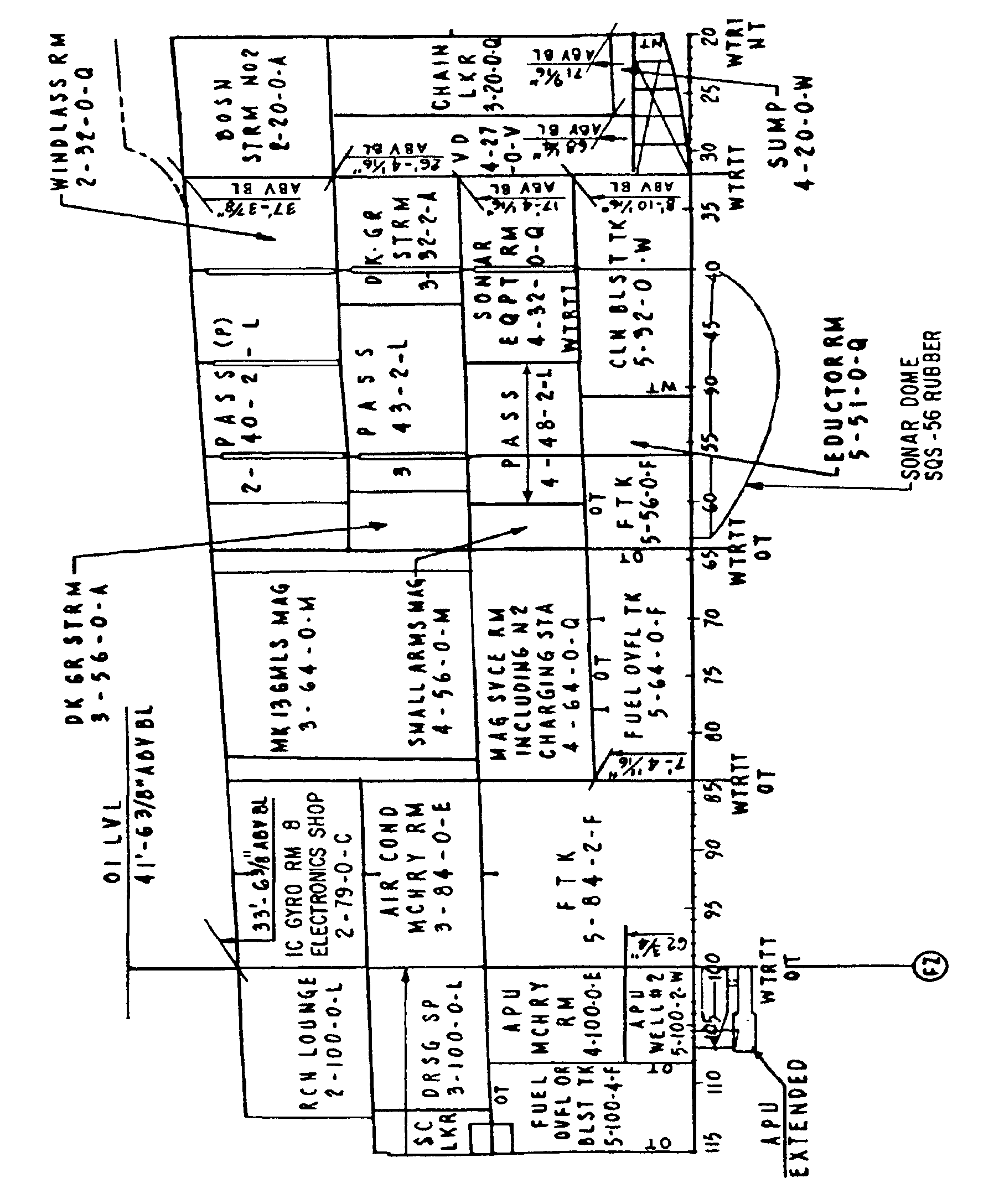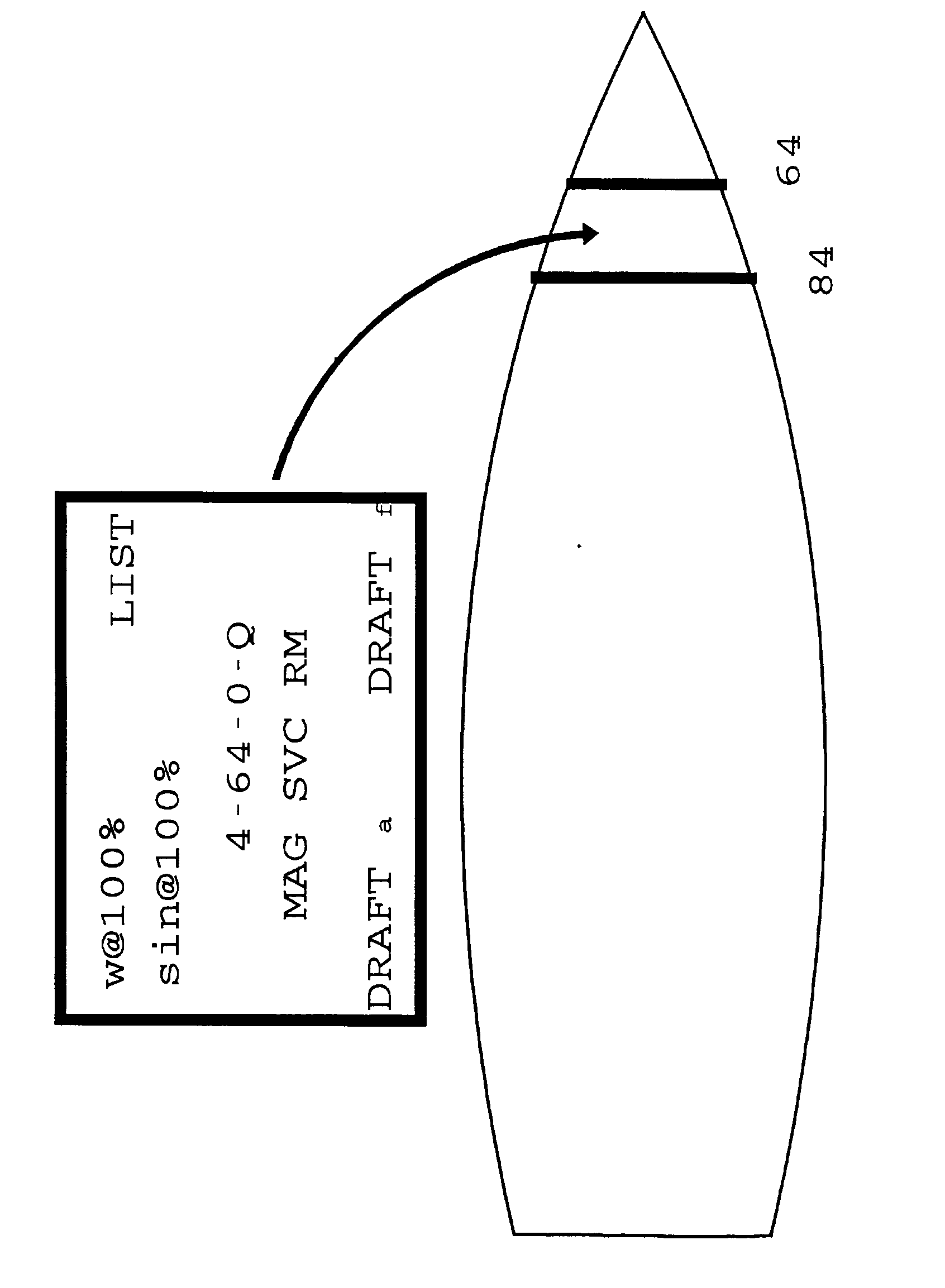UNDERWAY DRAFT REPORT

INTRODUCTION: When a ship is underway, it can be very difficult, often times impossible, to accurately read the draft marks at the waterline. Calculating the underway drafts is a simple procedure where fuel consumption is treated as a weight removal from within the storage tanks. A Trim Calculation Sheet can then be used to determine the change in forward and aft drafts due to parallel rise/sinkage and change in ship’s trim.

REFERENCES: Damage Control Book, Section II(a)

Ship’s Daily Fuel and Water Report

MATERIALS: Current and previous day’s fuel and water report

Trim Calculation Sheet

Damage Control Book, Section II(a)

Daily Draft Report

JOB STEPS: 1. Compare current and previous day’s fuel and water report, noting changes in liquid loading.

15 December 16 December

 Tank Capacity Actual Tank Capacity Actual 4-72-2-F 7875 7500 4-72-2-F 7875 4430 4-84-2-F 8925 8500 4-84-2-F 8925 8500 4-96-2-F 7875 7500 4-96-2-F 7875 7500 4-143-2-F 5250 5000 4-143-2-F 5250 4910 3-169-2-J 840 800 3-169-2-J 840 800

2. This report indicates that 3070 gallons of fuel were removed from tank 4-72-2-F. Knowing 322 gallons of fuel is one Long Ton, the liquid change can be converted to a weight change.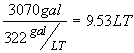3. Now that we have a weight change, the next step is to determine the Trimming Arm for the weight. Section II(a) of the Damage Control Book contains a Tank Capacity Data section which lists:

a) Each tank

b) The tank’s capacity in gallons and Long Tons

c) Conversion factors for LT, FT3, & Gallons

d) The tank’s vertical (KG) and longitudinal (LCG)

center of gravity

JOB STEPS: 3. The longitudinal center of gravity (LCG) is the distance (FT) from the center of gravity of the tank to the midships perpendicular. To get the Trimming Arm:

If the tank is forward of midships perpendicular, add LCF to the LCG distance.

If the tank is aft of midships perpendicular, subtract LCF from the LCG distance.

4. Complete the Trim Calculation Sheet using the weight, Trimming Arm, and Draft Diagram and Functions of Form information found in the damage control book, section II(a). Use of the Trim Calculation Sheet is discussed in Lesson 4.4, Trim and Draft.

5. The final result will be the calculated forward and aft drafts of your ship. These values will be used as the original drafts in the next day’s draft report.